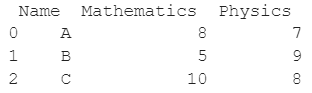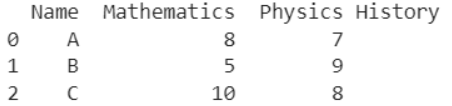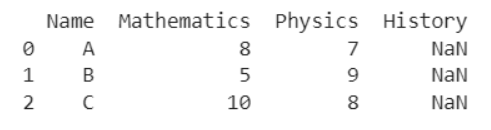# How To Add An Empty Column To A Pandas DataFrame?

A DataFrame is the primary data structure of the Pandas library and is commonly used for storing and working with tabular data in Python. A common operation that could be performed on such data is to add an empty column to an existing Pandas DataFrame in order to add more meaning to the information.

To start working with Pandas, we first need to import it by using the below statement:

``import pandas as pd``

## Running Example

Let us understand this operation with the help of an example. Consider the following DataFrame containing 3 students with names A, B, and C and their corresponding marks (out of 10) for two subjects, Mathematics and Physics.Code snippet for generating the above DataFrame:

``````import pandas as pd
# Dictionary for our data
data = {'Name' : ['A', 'B', 'C'], 'Mathematics' : [8, 5, 10], 'Physics' : [7, 9, 8]}
# DataFrame for the dictionary
df = pd.DataFrame(data)
# Printing the DataFrame
print(df)``````

Here, ‘data’ is a dictionary we created to initialize the DataFrame. For this, we use the .DataFrame() function of the Pandas library which takes the dictionary as an argument and returns the required DataFrame.

Now let us say that for some reason, we want to add another column with the label History to this DataFrame but we want the content of the column to be empty. The resulting output will look as follows :Let us look at different ways of performing this operation on a given DataFrame :

## Add an Empty Column by using simple assignment in Pandas Dataframe

In this method, we use simple assignment to add an empty column to an already existing Pandas DataFrame.

Let the column of interest be the one with the label as History here. df[‘History’] creates and references this newly created column and by virtue of simple assignment to an empty string, we are able to assign empty values to this newly created column with a label as History.

Let us look at the code and corresponding output for this method.

### Python 3 Code:

``````import pandas as pd
# Dictionary for our data
data = {'Name' : ['A', 'B', 'C'], 'Mathematics' : [8, 5, 10], 'Physics' : [7, 9, 8]}
# DataFrame for the dictionary
df = pd.DataFrame(data)
# Sort the DataFrame rows based on the values in the column labeled as Physics
df['History'] = ""
# Print the new DataFrame
print(df)``````

### Output :## Add an Empty Column by using DataFrame.assign() function in Pandas Dataframe

In this method, we use the DataFrame.assign() function to add an empty column to an already existing Pandas DataFrame.

Let the column of interest be the one with the label as History here. The label of the new column to be added is passed here as a parameter and an empty string is assigned to it.

This allows us to assign empty values to all cells in that column. By default the changes made in the DataFrame are not in place so reassignment is required.

Let us look at the code and corresponding output for this method.

### Python 3 Code :

``````import pandas as pd
# Dictionary for our data
data = {'Name' : ['A', 'B', 'C'], 'Mathematics' : [8, 5, 10], 'Physics' : [7, 9, 8]}
# DataFrame for the dictionary
df = pd.DataFrame(data)
# Sort the DataFrame rows based on the values in the column labeled as Physics
df = df.assign(History = "")
# Print the new DataFrame
print(df)``````

### Output :## Add an Empty Column by using DataFrame.reindex() function in Pandas Dataframe

In this method, we use the DataFrame.reindex() function to add an empty column to an already existing Pandas DataFrame.

Let the column of interest be the one with the label as History here. The labels of all columns to be present in the updated DataFrame are passed as a parameter here, this also includes the History column here.

This allows us to assign empty values to all cells in that column. By default the changes made in the DataFrame are not in place so reassignment is required.

Here, NaN stands for Not a Number and is equivalent to an empty value in Python. Let us look at the code and corresponding output for this method.

### Python 3 Code :

``````import pandas as pd
# Dictionary for our data
data = {'Name' : ['A', 'B', 'C'], 'Mathematics' : [8, 5, 10], 'Physics' : [7, 9, 8]}
# DataFrame for the dictionary
df = pd.DataFrame(data)
# Sort the DataFrame rows based on the values in the column labeled as Physics
df = df.reindex(columns = ['Name', 'Mathematics', 'Physics', 'History'])
# Print the new DataFrame
print(df)``````

### Output :## Add an Empty Column by using DataFrame.insert() function in Pandas Dataframe

In this method, we use the DataFrame.insert() function to add an empty column to an already existing Pandas DataFrame.

Let the column of interest be the one with the label as History here. The first parameter is the index at which the new column needs to be added, here 3.

The second parameter is the label of the new column to be added, here History. The third parameter is set as an empty string.

This allows us to assign empty values to all cells in that column. By default the changes made in the DataFrame are in place so reassignment is not required.

Let us look at the code and corresponding output for this method.

### Python 3 Code :

``````import pandas as pd
# Dictionary for our data
data = {'Name' : ['A', 'B', 'C'], 'Mathematics' : [8, 5, 10], 'Physics' : [7, 9, 8]}
# DataFrame for the dictionary
df = pd.DataFrame(data)
# Sort the DataFrame rows based on the values in the column labeled as Physics
df.insert(3, 'History', "")
# Print the new DataFrame
print(df)``````

### Output :## Conclusion

In this topic, we have learned to add an empty column to an existing DataFrame, following a running example of test scores of students in different subjects, thus giving us an intuition of how this concept could be applied in real-world situations. Feel free to reach out to info.javaexercise@gmail.com in case of any suggestions.

Trending